## Tangent Vector

For a curve with Position Vector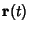, the unit tangent vector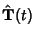is defined by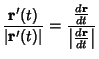(1)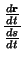(2)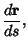(3)

where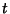is a parameterization variable and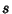is the Arc Length. For a function given parametrically by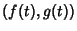, the tangent vector relative to the pointis therefore given by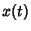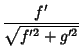(4)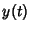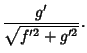(5)

To actually place the vector tangent to the curve, it must be displaced by. It is also true that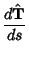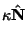(6)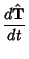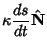(7)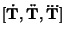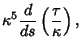(8)

where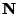is the Normal Vector,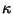is the Curvature, and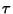is the Torsion.

See also Curvature, Normal Vector, Tangent, Tangent Bundle, Tangent Plane, Tangent Space, Torsion (Differential Geometry)

References

Gray, A. Tangent and Normal Lines to Plane Curves.'' §5.5 in Modern Differential Geometry of Curves and Surfaces. Boca Raton, FL: CRC Press, pp. 85-90, 1993.